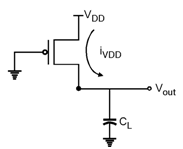Home > Digital CMOS Design > CMOS Inverter > Dynamic Power Consumption

CMOS-Inverter

Dynamic Power Consumption : In an inverter the capacitor CL is charged through the PMOS transistor, and hence some amount of energy is taken from the power supply. The some part of the energy is dissipated in PMOS and some is stored on the capacitor. Further, in high to low transition the capacitor is discharged and the stored energy is dissipated in the NMOS device. During low to high transition the load capacitance CL is charged. Hence the energy taken from the power supply to charge this capacitor .Fig-Dynamic-Power-Consumption

During low to high transition the load capacitance CL is charged. Hence the energy taken from the power supply to charge this capacitor is given as,

Energy taken from supply = 0 i(t) * VDD * dt = VDD 0 CL * dvoutdt * dt

= CL VDD VDD0 dvout = CL VDD2

Also, the energy stored on the load capacitor is given as,

Energy stored on capacitor = 0 i(t) * vout * dt = 0 CL dVoutdt Vout * dt

= CL VDD0 vout * dvout = CLVDD22

This results shows that the energy taken from the supply is CLVDD2 where as the energy stored on the capacitor is equal to CLVDD22 .

This shows that, the half energy is supplied by the power supply and stored in CL and other half is dissipated in pMOS device. Thus, in each switching cycle which consist of low-to-high to high-to-low transition a fixed amount of energy equal to CLVDD2 is taken,

Hence, If a gate is switched on and off 'f' times then the power consumption is given, by

Pdynamic = CLVDD2 * f

Hence, the dynamic power dissipation of the CMOS gate is depends upon the power supply voltage, the capacitance of the gate connected at the load and the switching frequency of the inputs.